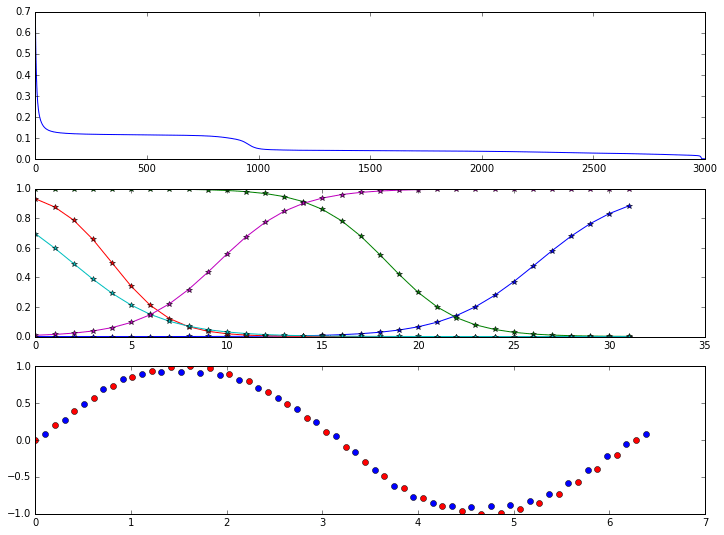# A Neural Network in Python, Part 2: activation functions, bias, SGD, etc.This is Part 2 of A Neural Network in Python, which was a very simple neural network to learn the XOR function. This part builds on that example to demonstrate more activation functions, learning a simple math function, adding a bias, improvements to the initial random weights, stochastic gradient descent, mean square error loss function,…